# ode45()

ode45() 算法是 MATLAB 中专门用于求解常微分方程（Ordinary differential equations，ODE）的函数，它在求解微分方程时的步长是变步长的，使用也是 RK 算法。

45 代表了 ode45() 使用 4 阶- 5阶 RK 算法。其中，4 阶方法用于提供 ODE 的候选解，5 阶方法用于控制误差，整体截断误差为 $\Delta x^5$。

# Runge-Kutta 算法

RK 算法是德国数学家 Runge 和 Kutta 在 1900 年前后提出的一种高精度求解微分方程的数值算法。该算法间接使用 Taylor 级数展开构造高精度数值方法，利用函数 $f$ 在若干点的值的线性组合代替 $f$ 的导数，再利用 Taylor 级数展开方法确定其中系数。

\left \{\begin{matrix} \begin{aligned} &\boldsymbol{\dot{y}} = f(t,\boldsymbol{y}),\\ &\boldsymbol{y}(t_0) = \boldsymbol{y}_0, \end{aligned} \end{matrix} \right.

\left \{\begin{matrix} \begin{aligned} &k_1 = f(t(k) ,\boldsymbol{y}(k)), \\ &k_2 = f(t(k) + h/2,\boldsymbol{y}(k) + k_1 h/2), \\ &k_3 = f(t(k) + h/2,\boldsymbol{y}(k) + k_2 h/2), \\ &k_4 = f(t(k) + h ,\boldsymbol{y}(k) + k_3 h), \\ &\boldsymbol{y}(k+1) = \boldsymbol{y}(k) + h(k_1+2k_2+2k_3+k_4)/6, \\ &\boldsymbol{y}(0) = \boldsymbol{y}_0. \end{aligned} \end{matrix} \right.

# RK 算法程序

%--- RK 算法 ---%
% ufunc - 微分方程组的函数名
% y0 - 初始值
% h - 步长
% a - 初始时间
% b - 末端时间
function [x,y]=runge_kutta1(ufunc,y0,h,a,b)
% 步数
n = floor((b-a)/h);
% 时间起点
x(1) = a;
% 初始值
y(:,1)=y0;

% 算法开始
for iter=1:n
% 时间变量
x(iter+1) = x(iter)+h;
% 开始迭代
k1 = ufunc(x(iter),       y(:,iter));
k2 = ufunc(x(iter) + h/2, y(:,iter) + h*k1 / 2);
k3 = ufunc(x(iter) + h/2, y(:,iter) + h*k2 / 2);
k4 = ufunc(x(iter) + h,   y(:,iter) + h*k3 );
% 得到结果
y(:,iter+1) = y(:,iter) + h * (k1 + 2*k2 + 2*k3 + k4) / 6;
end

end


# 仿真

\left \{\begin{matrix} \begin{aligned} \dot{y}_1 &= y_2 y_3, \\ \dot{y}_2 &= -y_1 + y_3, \\ \dot{y}_3 &= -0.51 y_1 y_2, \\ \boldsymbol{y} &= (y_{10},y_{20},y_{30}) = (1,1,3). \end{aligned} \end{matrix} \right.

## 仿真代码

clc;clear;close all;

%% 主函数
% 时间变量
t0 = 0;
tf = 1000;
% 初值
y10 = 1;
y20 = 1;
y30 = 3;
y = [y10,y20,y30];
% RK 算法步长
h = 0.25;
% 对该微分方程组用ode45和自编的龙格库塔函数进行比s较，调用如下：
% ode45()函数
tic;
[T,F] = ode45(@fun,[t0 tf],y);
time_record_ode = toc;
toc;

% 自编RK函数
tic;
[T1,F1]=runge_kutta1(@fun,y,h,t0,tf);
time_record_rk = toc;
toc;

%% 画图
figure('color',[1 1 1],'position',[600,100,500*1.5,416*1.5]);
subplot(121);
plot(T,F,'LineWidth',1.5);
title(['ode45','($t_f$=',num2str(tf),')'],'Interpreter','Latex');
set(gca,'FontSize',15,'FontName','Times New Roman','LineWidth',1.5);

subplot(122);
plot(T1,F1,'LineWidth',1.5);
title(['RK4','($t_f$=',num2str(tf),'),($h$=',num2str(h),')'],'Interpreter','Latex');
set(gca,'FontSize',15,'FontName','Times New Roman','LineWidth',1.5);

% 保存数据
str = ['tf_',num2str(tf),'_h_',num2str(h),'.mat'];
str_fig = ['tf_',num2str(tf),'_h_',num2str(h),'.jpg'];
save(str);
saveas(gcf,str_fig)
%% 子函数部分
% 微分方程
function dy = fun(t,y)
dy = zeros(3,1);%初始化列向量
dy(1) = y(2) * y(3);
dy(2) = -y(1) + y(3);
dy(3) = -0.51 * y(1) * y(2);
end

%--- RK 算法 ---%
% ufunc - 微分方程组的函数名
% y0 - 初始值
% h - 步长
% a - 初始时间
% b - 末端时间
function [x,y]=runge_kutta1(ufunc,y0,h,a,b)
% 步数
n = floor((b-a)/h);
% 时间起点
x = zeros(n,1);
x(1) = a;
% 初始值
y(:,1)=y0;

% 算法开始
for iter=1:n
% 时间变量
x(iter+1) = x(iter)+h;
% 开始迭代
k1 = ufunc(x(iter),       y(:,iter));
k2 = ufunc(x(iter) + h/2, y(:,iter) + h*k1 / 2);
k3 = ufunc(x(iter) + h/2, y(:,iter) + h*k2 / 2);
k4 = ufunc(x(iter) + h,   y(:,iter) + h*k3 );
% 得到结果
y(:,iter+1) = y(:,iter) + h * (k1 + 2*k2 + 2*k3 + k4) / 6;
end

end


## 不同终端时间下的算法对比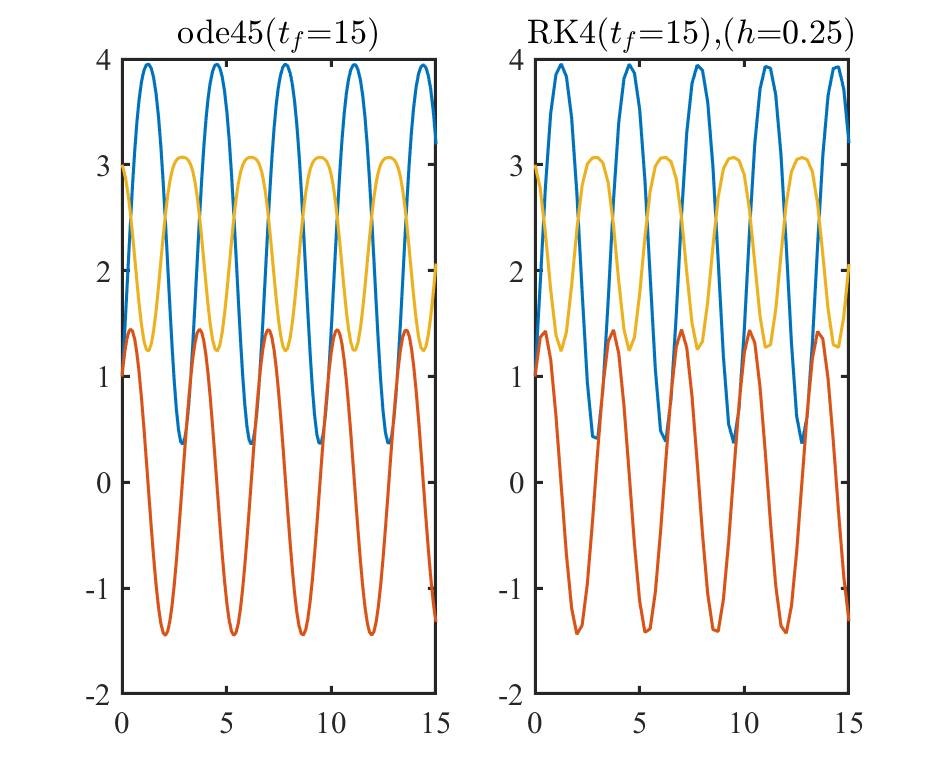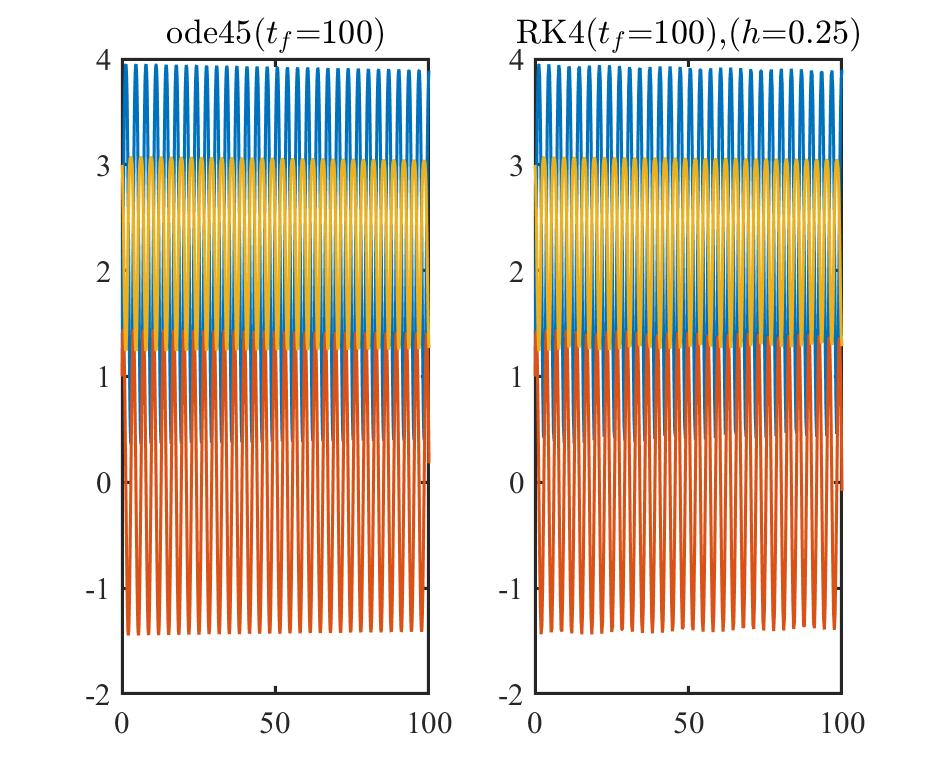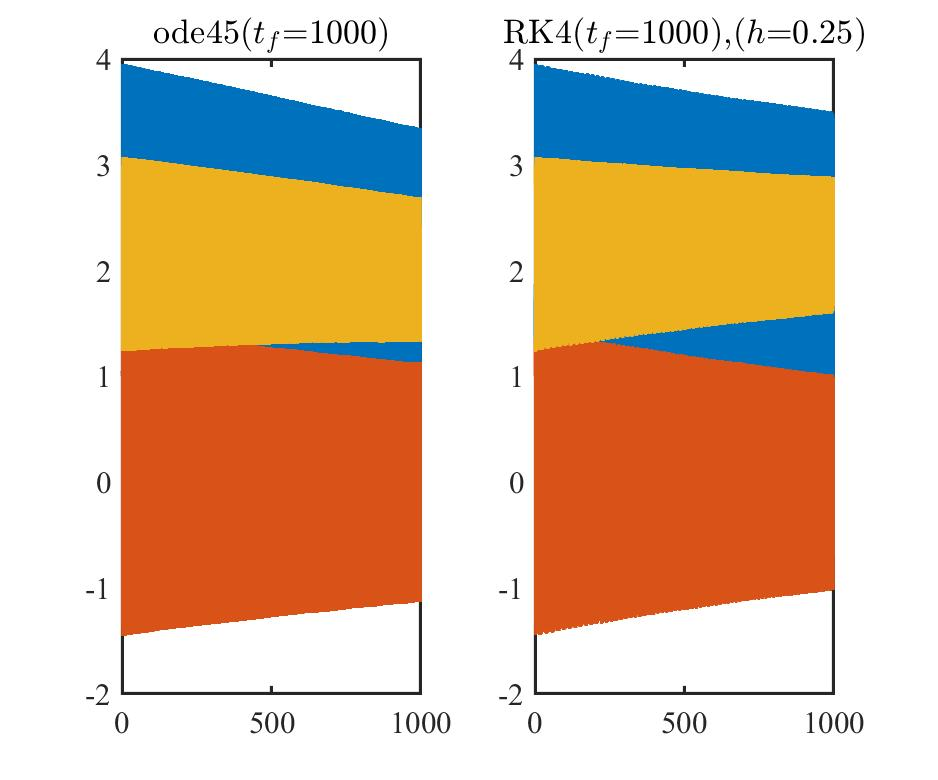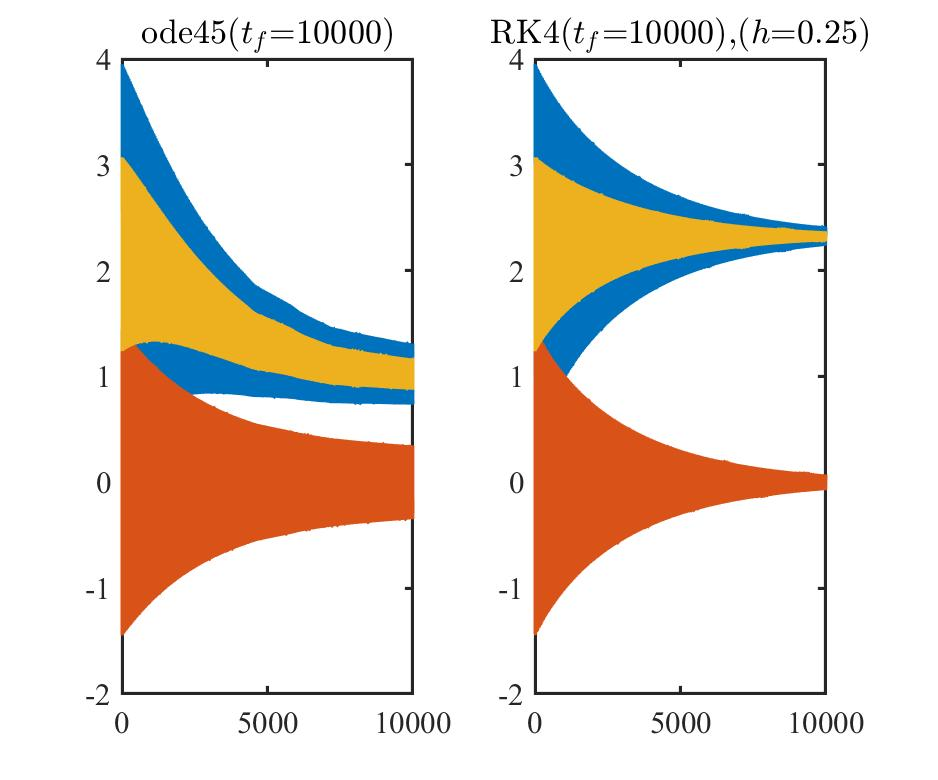$t_f=15$ $t_f=10^2$ $t_f=10^3$ $t_f=10^4$ $t_f=10^5$
ode45() 0.000863 0.002703 0.020696 0.165518 1.204862
RK4() 0.000448 0.001650 0.015817 0.136802 1.336549

## 不同步长下的算法对比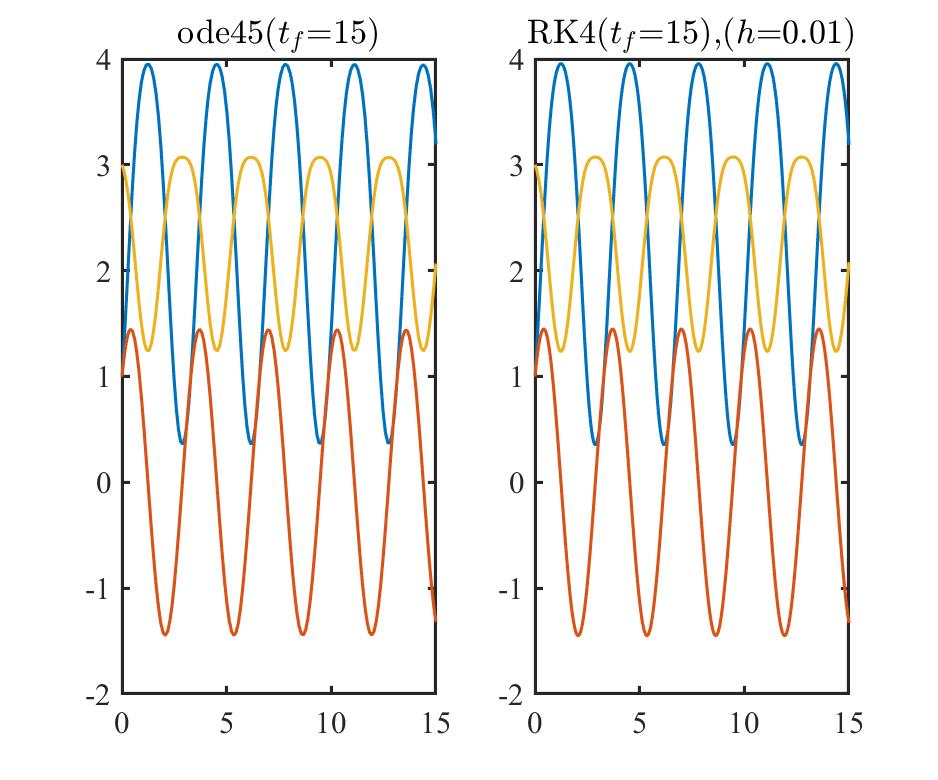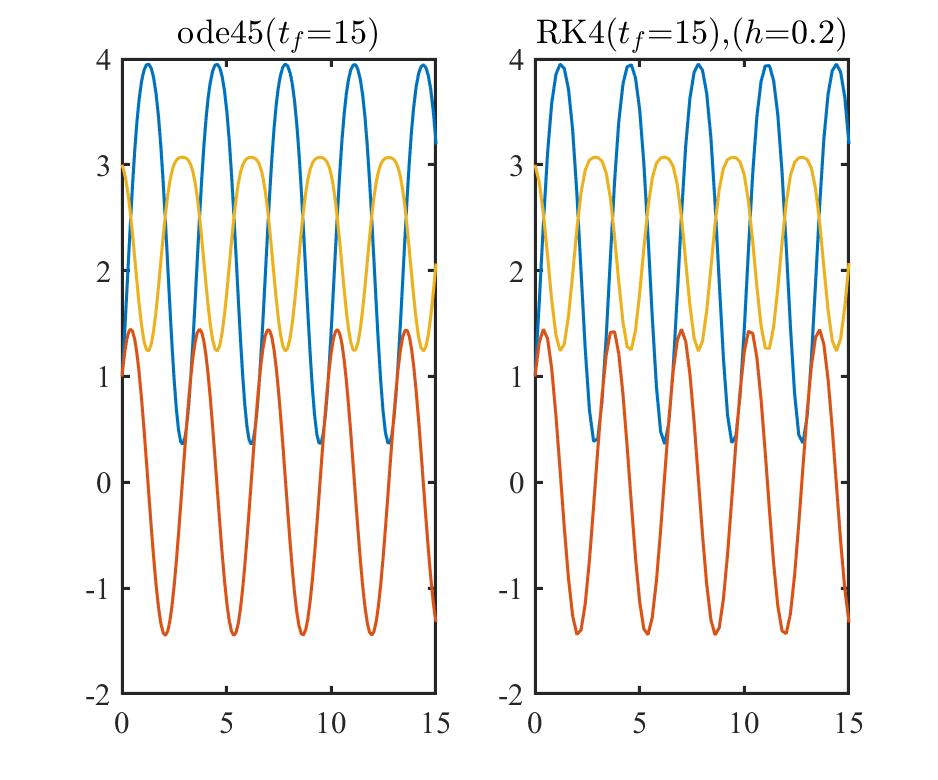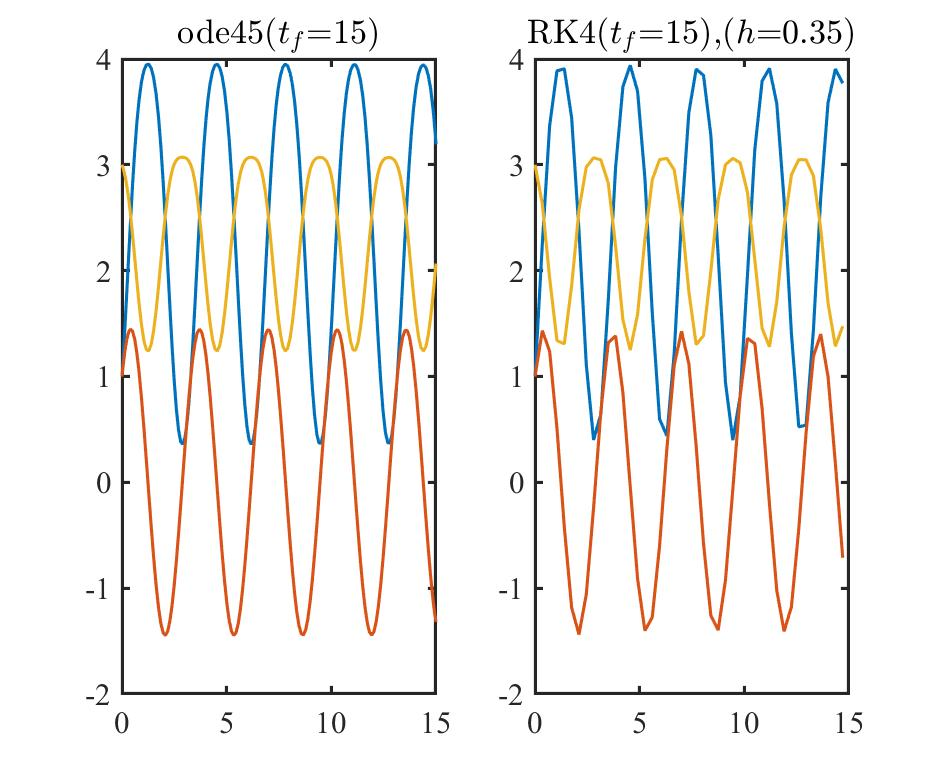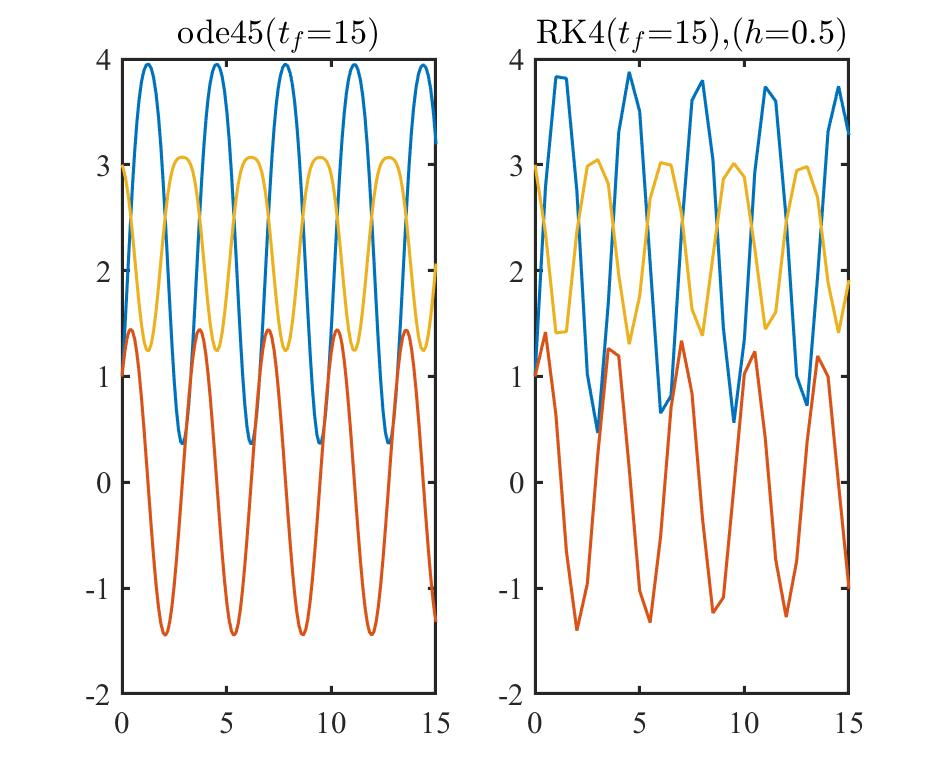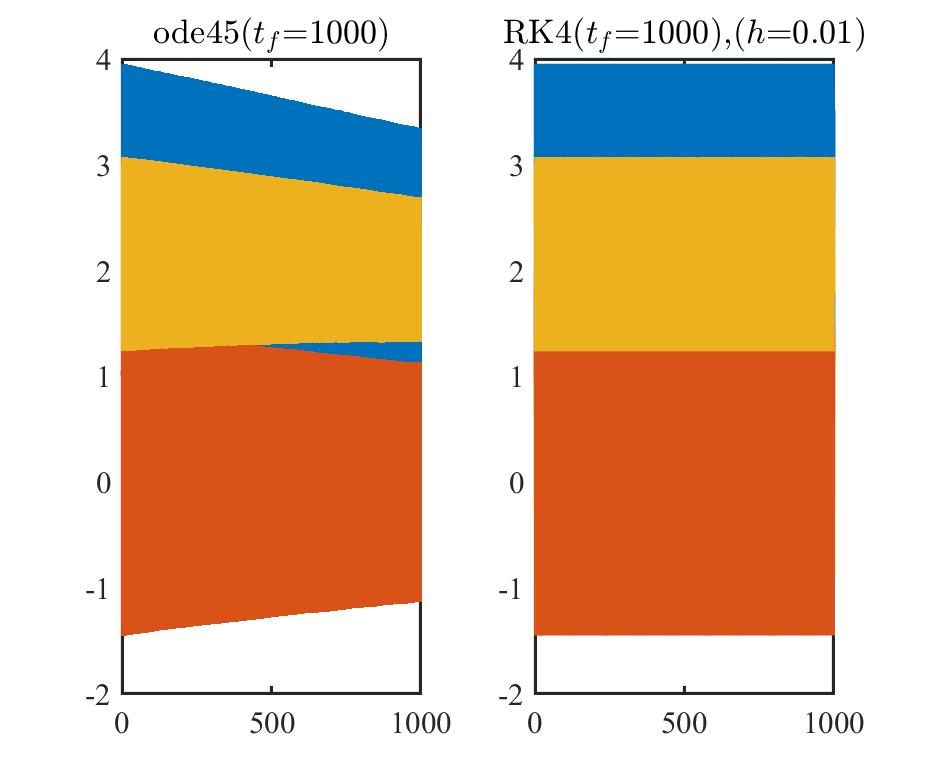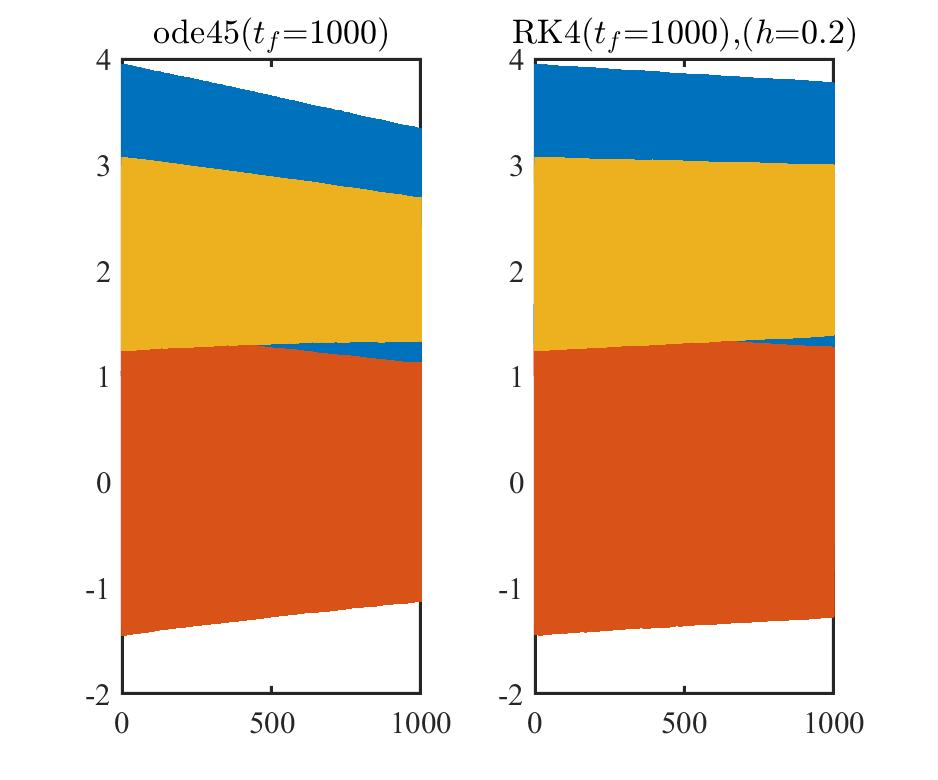$t_f=15$ $h=0.01$ $h=0.10$ $h=0.20$ $h=0.25$ $h=0.35$ $h=0.50$
ode45() 0.000795 0.000781 0.000881 0.000863 0.000992 0.000870
RK4() 0.005705 0.000901 0.000493 0.000448 0.000380 0.000251
$t_f=10^3$ $h=0.01$ $h=0.10$ $h=0.20$ $h=0.25$ $h=0.35$ $h=0.50$
ode45() 0.021820 0.020883 0.020322 0.020696 0.020698 0.020758
RK4() 0.343084 0.035581 0.017536 0.015817 0.011288 0.007863

# 结论

• 在计算时间短的微分方程求解问题中，使用自编 RK 算法的计算精度与使用 ode45() 计算精度相近，计算效率更高；
• 在计算时间长的微分方程求解问题中，建议使用 ode45() 会得到更好的结果；
• RK 算法中，计算步长和计算时间区间是需要协调好的重要变量，把这 2 个变量设置好，计算精度和计算效率才会更加优越。